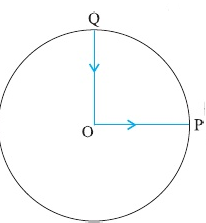## Question

### Solution

Correct option is

s

Let n seconds be the time taken by the stone to reach the ground. The distance covered in the last second = distance covered in n seconds – distance covered in (n – 1) seconds.

The distance covered in the first 3 secondsIt is given thatwhich gives n = 5 s.

#### SIMILAR QUESTIONS

Q1A cyclist starts from the centre O of a circular track of radius r = 1 km, reaches the edge P of the track and then cycles along circumference and stops at point Q. if the total time take is 10 min, what is the average velocity of the cyclist?

Q2

Give the speed-time graph of the motion of a car. What is the ratio of the distance travelled by the during the last two seconds to the total distance travelled in seven seconds?Q3

Two balls of masses m1 and m2 are thrown vertically upward with the speed u. If air resistance is neglected, they will pass through their point of projection in the downward direction with a speed

Q4

A train covers half of its journey with a speed of 20 ms–1 and the other half with a speed of 30 ms–1. The average speed of the train during the whole journey is

Q5

The displacement y (in metres) of a body varies with time t (in seconds) asHow long does the body take to come to rest?

Q6

A body goes from A to B with a velocity of 40 kmh–1 and returns from Bto A with a velocity of 60 kmh–1. What is the average velocity of the body during the whole journey?

Q7

The area under the velocity-time graph between any two instants t = t1and t = t2 gives the distance covered in timeQ8

A particle is given a displacement of 4 m in the x-y plane. If the x-component of the displacement vector is 2 m, the y-component will be